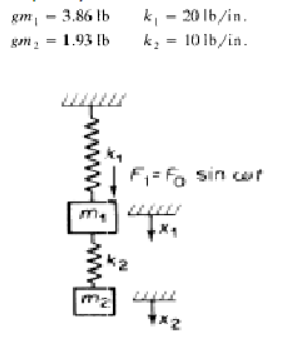Home / Expert Answers / Mechanical Engineering / for-the-system-shown-derive-equations-of-motion-and-find-the-natural-frequencies-the-eigen-modes-pa246

# (Solved): For the system shown, derive equations of motion, and find the natural frequencies, the eigen modes ...

For the system shown, derive equations of motion, and find the natural frequencies, the eigen modes and the amplitudes of displacements under zero initial conditions as functions of frequency of external force ????.

gm1= 3.86 lb
gm2 =1.93 lb

k1= 20 lb/in
k2= 10 lb/inWe have an Answer from Expert